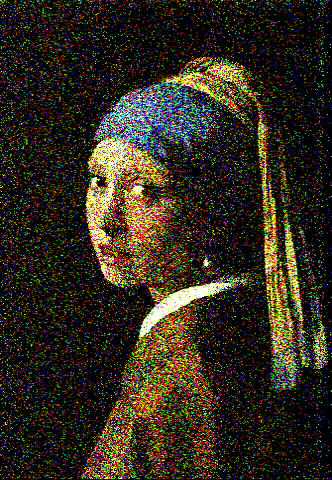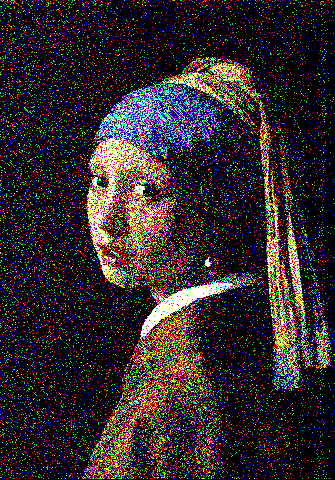# Background

I want to display rich, vibrant images on my theoretical fancy custom display, but due to budget constraints, it displays everything in 3-bit colour! Whilst Regular Dithering would work, I don't want to sacrifice resolution, so I would like my images to be dithered through time!

# Specs

Provided an Input Image, I would like you to show me several 3-bit color versions of that image, such that the average colour of all images is the original image.

## I/O

You must take an image as input.

You may either animate the image (Return a GIF, Video, or on the screen), or take time as an additional input. If time is taken as input, the output must be Deterministic for a given image and time, and must return as a static image.

## ExampleFrom Wikimedia CommonsA lot of quality was lost between recording the output and converting it to a gif, thus a still has also been provided

## Victory

The victory condition is , as per previously stated budget constraints.

# Final Notes

• What does 3-bit color means? How should I calculate average color? Will input image always use RGB color space?
– tsh
Dec 1, 2021 at 5:22
• I think these would be helpful. Would you mind to edit these into the question itself?
– tsh
Dec 1, 2021 at 8:37
• @theorist As stated in I/O, the output must either be animated, such as the first option in the spoiler, or a static output for a given time input. Both the example images provided are 3-bit (Ignoring the aliasing artifacts in the GIF) Dec 1, 2021 at 9:19
• You should have further constraints on what counts as dithering, as otherwise something like f=lambda i,t:[int(p>t%255)for p in i] is a valid submission Dec 1, 2021 at 10:24
• @AnttiP I did consider that as a solution, and whilst it's not the most visually interesting answer, it's not that much more complex than p>rand(), and enforcing interesting solutions is difficult. Dec 1, 2021 at 11:03

# Wolfram Language (Mathematica), 24 bytes

Sign[#-Mod[1##,1]]&


Try it online!

Input [image,time], where image is a 3-channel RGB Image, and outputs a frame.

A fairly straightforward approach: output whenever the floor of the accumulated value increases. Mathematica's Images support arithmetic and can contain values of any size, but are clamped between 0 and 1 when displayed.

For the image in OP, a GIF output of t=1,2,...,255 can be viewed here (caution: blocks of flashing colors).
Averaging the frames:# Perl 5 -ap, 110 bytes

srand($F=1);$_=(map{splice@b,rand@b,1}@b=((1)x($o=int$_*8/256),(0)x(8-$o)))[$ENV{F}]for@F[4..$#F];$_="@F\n"


Can't try it online!

...but instead you can create an 8 frames animated GIF image with:

echo 'srand(7);$F=1;$_=(map{splice@b,rand@b,1}@b=((1)x($o=int$_*8/256),(0)x(8-$o)))[$ENV{F}]for@F[4..$#F];$_="@F\n"' > program.pl
for f in {0..7};do cat img.ppm|F=$f perl -ap program.pl > img-frame$f.ppm;done  #~10sec

The program.pl reads the one line input (the example image of this challenge converted to the Plain PPM format) from STDIN and the frame number 0-7 (the time) from the environment variable F. The above commands works with bash shell, wget, the convert program from ImageMagick and xdg-open or any image viewer capable of viewing animated GIF images. To change to 16 frames, replace 7 with 15 and the two 8's with 16. Animated GIF image: https://drive.google.com/file/d/1s5WDGjwKLowdmildkYxUWGOWpRjS-Av6/view?usp=sharing